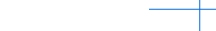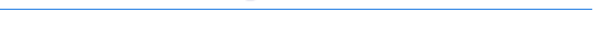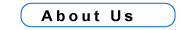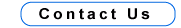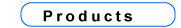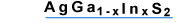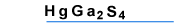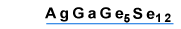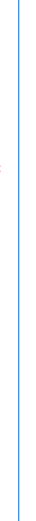AgGaGeS4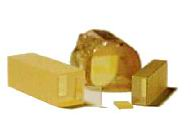Structural and Physical Properties
 Crystal symmetry and class Orthorhombic, C2v ( mm2 ) Space group C192v Cell parameters(A) a = 12.028, b = 22.918, c = 6.874 Melting point (oC) 835 ± 10 Optical homogeneity Dn < 1*10-4 Density (gram/cm3) 3.80 Absorption coefficient (cm -1) < 0.005Optical and NLO PropertiesOptical transmission ( mm ) 0.5 to 11.5 Fundamental absorption edge ( mm ) 0.47 Energy gap (eV) 2.64 Surface damage threshold ( t = 10 ns, l = 1.06 mm) - 50 MW/cm2Dispersion of refractive indices(Experimental values)l (mm) nx ny nz 0.5 2.4377 2.5128 2.5125 0.6 2.3706 2.4355 2.4362 0.8 2.3151 2.3723 2.3733 1.064 2.2866 2.3411 2.3421 2.0 2.2599 2.3112 2.3144 4.0 2.2497 2.2985 2.3013 10.6 2.2139 2.2472 2.2448 11.5 2.2068 2.2369 2.2324
Nonlinear optical coefficients ( pm/V ):d31 = ± 15; d32 = ± 8.0; d33 = ± 8.0. X - c, Y - b, Z - a; At l = 1.064 mm 2V = 164.8o
dij defined in the abc frame (mm2 point group, c - polar axis)

Dispersion of refractive indices ( Sellmeier equations, l in mm )n2x = 11.14357 - 0.16361483 / ( 0.06081829 - l2 ) - 24267.167 / ( 4000 - l2 );
n2y = 11.94768 - 0.18261602 / ( 0.06821353 - l2 ) - 19915.432 / ( 3000 - l2 );
n2z = 12.64467 - 0.17736979 / ( 0.07099873 - l2 ) - 21966.269 / ( 3000 - l2 ).

Types of possible nonlinear processes in the principal planes
of the AgGaGeS4 and effective nonlinearity deff
 XY plane YZ plane XZ plane q < V oe - e, eo - e, deff = 0 oe - o, eo - o, deff = 0 oo - e, deff = d32 cosq; oo - e, deff = d31 sinj ee - o, deff = d31 sin2q + d32 cos2q oe - e, eo - e, deff = 0

Application

The AgGaGeS4 crystals can be used for frerquency doubling and three-frequency mixing. Parametric oscillators and amplifiers based of AgGaGeS4 can generate continuously tunable radiation in the spectral range from 1 to 11 mm with suitable pump laser.